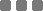## Tuesday, March 17, 2015

### JavaScript: Object Literal Pairs Basic Sorting (Number and Letter)

In JavaScript, there's `sort([compareFunction])` method that we can utilize to sort an array. By default, if we do not provide the comparing function, just `sort()`, the sorting will be based on the input strings given. Read more at Mozilla Developers Network documentation.

Let's get right on it
For instance, we have this object literal pairs:

We cannot sort those `[property]: [value]` pairs directly. There's no direct built-in method in JavaScript for that (yet). We need to convert the object into an array first, then we can sort it.
I typed a basic application (in JavaScript) to sort the object input by number or letter.
In this example, there are two options for the function.

What I did in this function example are:
1. The object `{ [property]: [number_value], [property]: [number_value], ... }` is transformed into an array. I need to put the sorting reference in front of the array element, therefore:
• for number-based sorting, the array will consist of:
`[ number_value|property, number_value|property, ...]`
To make it simple, the "keyword" for this sorting option is `"number"`.
• For letter-based sorting, the array will consist of:
`[ property|number_value, property|number_value, ...]`
This sorting option has `"letter"` for the keyword.
2. Then the sorting starts using either `sort([compareFunction])` (for number-based) or just `sort()` (for letter-based).
3. Continued by creating new object literal which has the new sorted `[property]: [number_value]` pairs from the earlier sorted array.
Since this object example has `strings: numeral` pairs, I use `RegEx` to separate each of the earlier sorted array elements into object pairs.

Here's the complete function and usage
I put comments in this, I hope you can follow the story line.
The `function` must be declared on top before we can invoke it. At the bottom, you'll see how to "call" it.
Updated

On Chrome, you can open the JavaScript `console` ► copy-paste this example ► press [enter] or [return] ► you'll see the result: the first one is the original object and the second is the sorted object.
Omit the very last comment `//` sign to see both results. Or switch it to see only either one of them.

I only put additional `compareFunction`, that is:
only for number-based sorting. The `parseFloat` method is to extract only the `number_value` from this pattern: `number_value|property`, which is a number with decimal point.
If it's strictly integer, we can use `parseInt(string, 10)`. The 10 there is the number base, decimal, base 10.

This will sort from the smallest to the largest/highest. To reverse the sorting, we can use `reverse()` method for the array. As in:

About the `regular expression`
As you can see there, I typed `ar[j].match(/[a-zA-Z_]+/g)` for extracting the letters-only from the array element `ar[j]`. The `[a-zA-Z]` is to match the Latin alphabet, and additional underscore [_] is for filtering my own object property keyword. I put A_ten, B_six, C_twenty, etc. There's that underscore, so I put it there on the pattern matching.

For the numeral matching, I typed `/[.-\d]+/g`. The `\d` will match all the digit, and the additional dot [.] and dash [-] are for decimal point and negative number, respectively. The [+] plus there is for matching the repeated pattern.
We can also use parseInt or parseFloat for that purpose (extracting number). Since it's placed in front of the element.
But there's the other option which has the `number_value` behind the `property` letters. Therefore, to facilitate both options, I put only regular expression.

The `g` in all of them there is for matching globally.

About the `join`
On the "create new object literal" part, you can see I put `join("")` there. It's because the output of `match` method is an array. Therefore, I need the `join` method to convert it to "just" strings.
Then convert it to numeral type, using `Number()`, to be consistent with the original object value type.

About the object output on Chrome console
For the number-based sorting, you can see the outputs as:
• `Object {C_twenty: 20, B_six: 6, D_threeHundred: 300, A_ten: 10, E_minusEightOne: -81…}`
• `Object {E_minusEightOne: -81, B_six: 6, A_ten: 10, C_twenty: 20, F_eightyPointSix: 80.6…}`
The first one is the original object, and the second is the sorted object.

But, if it is expanded, each object will be re-sorted based on the property keyword first letter. That's the built-in "neatifier" in Chrome.
The "actual" output is at the first line, before it's expanded.

In conclusion, hehe...
This one was a browser console doodle. Pretty [adjective_here], I think. Especially for multiple fields, like multidimensional array in JSON response. Nevertheless, that's kinda the "basic" of it. For pairs. Sort of. Well, that's how I interpreted it. *horse grin*

Useful Q&A on Stack OverflowJavaScript: Object Literal Pairs Basic Sorting (Number and Letter)
https://monkeyraptor.johanpaul.net/2015/03/javascript-object-literal-pairs-basic.html